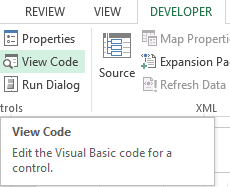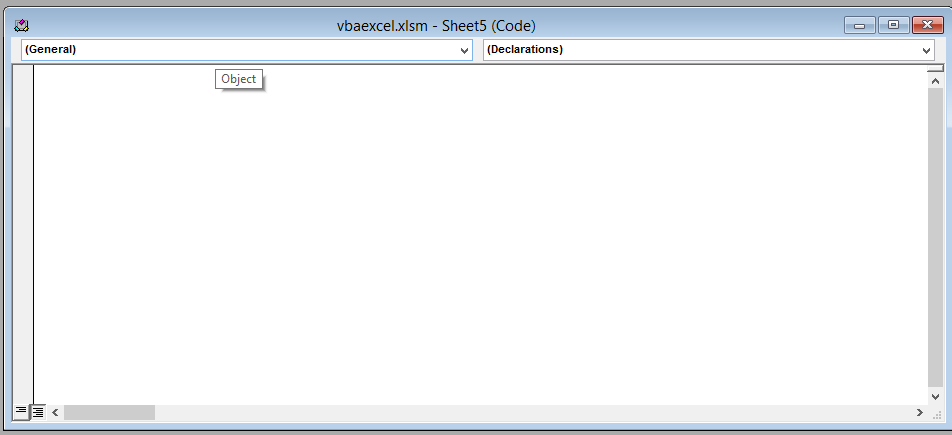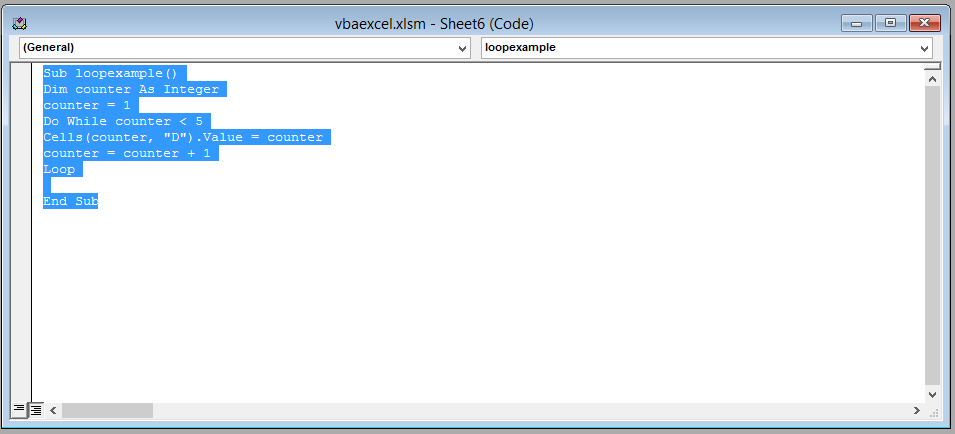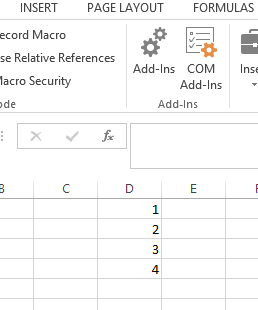#### Do While Loop in Excel Vba

In this Excel VBA tutorial lesson, you are going to learn about programming DO WHILE loops. First, you are going to learn what loops are and why they are necessary. Then you are going to learn how to use a DO WHILE LOOP.

## Types of VBA loops

Note: In Excel VBA there are two types of DO LOOPS: DO WHILE and DO UNTIL. Our focus will be on the DO WHILE loop in this article.

Consider the following code:

```Dim numbcount As Integer
numbcount = 1 + 2 + 3 + 4 + 5 + 6 + 7 + 8 + 9 + 10```

This code is used to add the numbers from 1 to 10. The result is then stored in the variable numbcount. Now this looks pretty easy and simple right now. But now suppose instead of the first ten number you want to add numbers 1 to 1000, now are you going to write all the numbers and then add them? Of course you can, but that would become tedious. You use the handy programming tool called “Loop”.

Loop as the names sound is something that goes on and on until you tell it to stop (this is called a “condition”). You set a starting number for your loop, an end condition, and a way to get from the starting number to the end condition. In VBA, there are four types of loops to choose from: For loops, For Each loop, Do Loops, while wend loop and While loops. You will focus on the DO WHILE loop for now.

First let’s look at the syntax of the DO WHILE LOOP:

```Do While [CONDITION]
Loop```

Explanation: First you declare the DO WHILE loop by writing “Do While” followed by square brackets in which you write a condition. This condition will be used to end your DO WHILE loop.

Here is an example for you to understand the syntax better:

```Do While count<=5
Count=count+1
Loop```

Explanation: This code will increase the value of a variable called count by 1 and then store the value in the same variable count. This loop will keep on going again and again until the condition statement is true, meaning until the value of count is less than equal to 5. Now let’s see how to use the Do WHILE loop. Follow the steps below:

## The ribbon

Click on the Developer tab and select “View Code”.A new window (Visual Basic Editor) will open which will have a dialog box in the center. You will write the code in the dialog box.## The code

Write the following line of code in the dialog box.

```Sub loopexample()
Dim counter As Integer
counter = 1
Do While counter < 5
Cells(counter, "D").Value = counter
counter = counter + 1
Loop
```

After writing the code close the window by clicking on the cross(x) icon the the upper right side of the screen.

Explanation: In this code you have declared a variable “counter” of data type integer and has assigned the value 1 to it. You used the DO WHILE loop with the condition to count from 1 to 4 and store the value in cell D.After writing this code, close the window (visual basic editor) by clicking on the upper right cross button.

## The result

This is the result. You have now successfully used a DO WHILE loop.